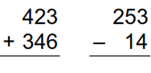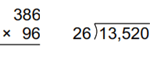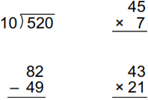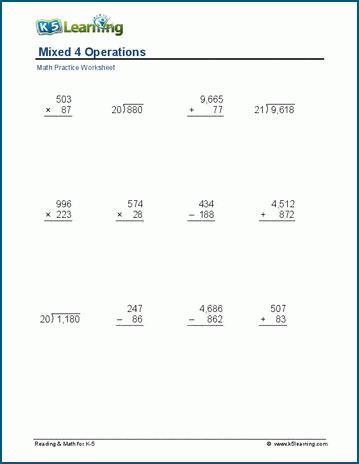# Mixed 4 operations worksheets

## Mixed practice

These worksheets provide a mix of multi-digit addition, subtraction, multiplication and division questions. Great practice for students who are doing well on basic skills with the 4 operations.

We also have mixed math facts worksheets and thousands of math worksheets by grade level.

Mixed addition and subtraction (2-digits)Mixed addition and subtraction (3 digits)
Mixed addition and subtraction (4 digits)

## Mixed multiplication and division

Mixed multiplication and division (2 digits)Mixed multiplication and division (3 digits)
Missing factors ___ x 12 = 600
Missing divisor and dividends 1,012 ÷___ = 44

## Mixed 4 operations

Mixed 4 operations (1-3 digits)Mixed 4 operations (2-4 digits)Sample mixed operations worksheet

What is K5?

K5 Learning offers free worksheets, flashcards and inexpensive workbooks for kids in kindergarten to grade 5. Become a member to access additional content and skip ads.